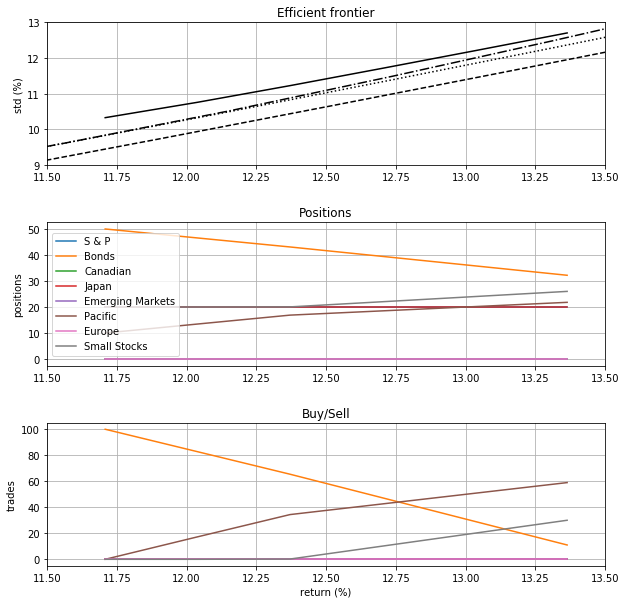Portfolio Optimization¶

This notebook can be run online without installing any packages. Just click the logo:to run it on binder.

See this case study for more details.

Import needed libraries¶

In :
import math
import numpy as np
import matplotlib.pyplot as plt
import pandas as pd

In :
In :

Classic Markowitz Portfolio Optimization¶

In the classic Markowitz optimal portfolio optimization (Markowitz, 1952), one seeks to determine

• $x$: a vector of porfolio positions,

that minimize the total portfolio variance given:

• $r$: the vector of expected returns,
• $Q$: the covariance matrix of the returns,
• $\mu$: the desired portfolio return.

The problem can be formulated mathematically as the Quadratic Program (QP): \begin{aligned} \min_{x} \quad & x^T Q x \\ \text{s.t.} \quad & r^T x = \mu \quad \sum_i{x_i} = 1 \end{aligned}

In this notebook we will show how one can setup and solve such portfolio optimization problems using the Optimize API.

Since we are using Python, we will take advantage of numpy and pandas to manipulate the data in this notebook. We will also use matplotlib to plot the data. The use of such libraries or even Python is not a requirement for using the API, which can be accessed using AJAX calls.

Data for the problem considered in this demo is taken from Elton and Gruber, 1995.

References:¶

1. Harry M. Markowitz, “Portfolio Selection”. Journal of Finance, 7(1): 77 - 91, 1952.
2. Edwin J. Elton and Martin J. Gruber, Modern Portfolio Theory and Investment Analysis. Fifth Edition, John Wiley and Sons, 1995.

Setup data using pandas¶

Consider the following set of assets with associated expected return and return stadard deviation given as percentages assembled as a pandas DataFrame:

In :
data = [
['S & P',14.0,18.5],
['Bonds',6.5,5.0],
['Japan',14.0,23.0],
['Emerging Markets',16.0,30.0],
['Pacific',18.0,26.0],
['Europe',12.0,20.0],
['Small Stocks',17.0,24.0]
]
assets = pd.DataFrame(data, columns=['Label', 'Return (%)', 'STD (%)'])
assets
Out:
Label Return (%) STD (%)
0 S & P 14.0 18.5
1 Bonds 6.5 5.0
3 Japan 14.0 23.0
4 Emerging Markets 16.0 30.0
5 Pacific 18.0 26.0
6 Europe 12.0 20.0
7 Small Stocks 17.0 24.0

The assets are associated with the following correlation coefficients:

In :
n = len(assets)
data = np.array([
[1,.45,.7,.2,.64,.3,.61,.79],
[.45,1,.27,-.01,.41,.01,.13,.28],
[.7,.27,1,.14,.51,.29,.48,.59],
[.2,-.01,.14,1,.25,.73,.56,.13],
[.64,.41,.51,.25,1,.28,.61,.75],
[.3,.01,.29,.73,.28,1,.54,.16],
[.61,.13,.48,.56,.61,.54,1,.44],
[.79,.28,.59,.13,.75,.16,.44,1]
])
cov = pd.DataFrame(data=data, columns=assets.Label.values, index=assets.Label.values)
cov
Out:
S & P Bonds Canadian Japan Emerging Markets Pacific Europe Small Stocks
S & P 1.00 0.45 0.70 0.20 0.64 0.30 0.61 0.79
Bonds 0.45 1.00 0.27 -0.01 0.41 0.01 0.13 0.28
Canadian 0.70 0.27 1.00 0.14 0.51 0.29 0.48 0.59
Japan 0.20 -0.01 0.14 1.00 0.25 0.73 0.56 0.13
Emerging Markets 0.64 0.41 0.51 0.25 1.00 0.28 0.61 0.75
Pacific 0.30 0.01 0.29 0.73 0.28 1.00 0.54 0.16
Europe 0.61 0.13 0.48 0.56 0.61 0.54 1.00 0.44
Small Stocks 0.79 0.28 0.59 0.13 0.75 0.16 0.44 1.00

Solve problem using the Optmize API¶

Before solving the problem we need to assemble the required data, that is the vector of expected returns $r$, which can be taken directly from the data:

In :
# vector of expected returns
r = assets['Return (%)'].values

the covariance matrix $Q$, which can be calculated from the given data as follows:

In :
# covariance matrix
sigmas = np.diag(assets['STD (%)'].values)
Q = sigmas @ cov.values @ sigmas

In the context of the classic Markowitz portfolio optimization we should also specify a desired expected return

In :
# expected return
mu = 14

and then select a portfolio corresponding to this return with the smallest possible variance.

The actual calculation of the optimal portfolio will be done on the cloud.

Submit problem to the api¶

We assemble all the data in an object and make an api call to get the optimal portfolio:

In :
# prepare data for submitting request to api
data = {
'Q': Q.tolist(),
'r': r.tolist(),
'mu': mu
}
sol = client.call('portfolio', data)

Once an optimal solution is calculated we append it to the dataframe.

In :
if sol['status']=='infeasible':
print('> Problem is infeasible')
assets['Optimal (%)'] = np.nan
else:
print('> Problem is feasible')
print('  * mu = {:.3f}%, std = {:.3f}% ({})'.format(mu, math.sqrt(sol['obj']), sol['status']))
pd.options.display.float_format = '{:,.1f}'.format
assets['Optimal (%)'] = 100*np.array(sol['x'])
assets
> Problem is feasible
* mu = 14.000%, std = 12.930% (optimal)
Out:
Label Return (%) STD (%) Optimal (%)
0 S & P 14.0 18.5 5.3
1 Bonds 6.5 5.0 37.0
3 Japan 14.0 23.0 6.9
4 Emerging Markets 16.0 30.0 -12.0
5 Pacific 18.0 26.0 33.7
6 Europe 12.0 20.0 -11.5
7 Small Stocks 17.0 24.0 44.2

By default, the solution vector adds to one, each number being interpreted as the fraction of the available budget that should be invested in order to realize the optimal portfolio. We use numpy to convert that to a percentage before displaying the solution.

Solution without short sales¶

The minimum variance portfolio that meets a required level of expected return is often one in which the positions can be negative. Negative positions are realized as short sales.

For example, in the problem solved above

In :
x = assets['Optimal (%)'];
print('{:.1f}%'.format(-x[x < 0].sum()))
27.1%

of the portfolio is comprised of short sales.

One can add constraints to the optimization problem in order to prevent short sales.

This can be done by adding the following option:

In :
# options
options = {
'short': False
}

and calling the API once again

In :
# prepare data for submitting request to api
data = {
'Q': Q.tolist(),
'r': r.tolist(),
'mu': mu,
'options': options
}
sol = client.call('portfolio', data)

The new solution

In :
if sol['status']=='infeasible':
print('> Problem is infeasible')
assets['No Shorts (%)'] = np.nan
else:
print('> Problem is feasible')
print('  * mu = {:.3f}%, std = {:.3f}% ({})'.format(mu, math.sqrt(sol['obj']), sol['status']))
assets['No Shorts (%)'] = 100*np.array(sol['x'])
assets
> Problem is feasible
* mu = 14.000%, std = 13.365% (optimal)
Out:
Label Return (%) STD (%) Optimal (%) No Shorts (%)
0 S & P 14.0 18.5 5.3 0.0
1 Bonds 6.5 5.0 37.0 30.9
2 Canadian 11.0 16.0 -3.6 0.0
3 Japan 14.0 23.0 6.9 2.7
4 Emerging Markets 16.0 30.0 -12.0 0.0
5 Pacific 18.0 26.0 33.7 32.1
6 Europe 12.0 20.0 -11.5 0.0
7 Small Stocks 17.0 24.0 44.2 34.4

is one without short sales:

In :
x = assets['No Shorts (%)']
print('{:.1f}%'.format(-x[x < 0].sum()))
0.0%

in which each every position is an asset purchase:

In :
print('{:.1f}%'.format(x.sum()))
100.0%

Note that the new portofolio is riskier than the optimal one with short sales.

Accounting for cashflow¶

Now suppose that one has an initial portfolio that they would like to rebalance, or buy or sell more assets.

In this case, one starts with an initial positions, for example:

In :
assets['Initial (%)'] = [20, 30, 0, 20, 0, 10, 0, 20]
assets
Out:
Label Return (%) STD (%) Optimal (%) No Shorts (%) Initial (%)
0 S & P 14.0 18.5 5.3 0.0 20
1 Bonds 6.5 5.0 37.0 30.9 30
2 Canadian 11.0 16.0 -3.6 0.0 0
3 Japan 14.0 23.0 6.9 2.7 20
4 Emerging Markets 16.0 30.0 -12.0 0.0 0
5 Pacific 18.0 26.0 33.7 32.1 10
6 Europe 12.0 20.0 -11.5 0.0 0
7 Small Stocks 17.0 24.0 44.2 34.4 20

An optimal portfolio will not change depending on whether one holds assets or not, so the answers obtained so far should not be affected by the existing holdings.

However, imagine that one has cash to invest and would like to know what is the best solution for investing the additional money without selling any of their assets.

One can take that scenario into account by adding an initial portfolio:

In :
# initial portfolio
x0 = assets['Initial (%)'].values/100

and the desired cashflow, say equivalent to 40% of the existing portfolio,

In :
# cashflow
cashflow = 40/100

along with the other data:

In :
# expected return
mu = 14

# options
options = {
'sell': False
}

# prepare data for submitting request to api
data = {
'Q': Q.tolist(),
'r': r.tolist(),
'mu': mu,
'x0': x0.tolist(),
'cashflow': cashflow,
'options': options
}
sol = client.call('portfolio', data)

The optimal solution now is in the form of additional buys that need to be realized within the specified cashflow to achieve the desired return:

In :
if sol['status']=='infeasible':
print('> Problem is infeasible')
assets['After Cashflow (%)'] = np.nan
else:
print('> Problem is feasible')
print('  * mu = {:.3f}%, std = {:.3f}% ({})'.format(mu, math.sqrt(sol['obj']), sol['status']))
x = np.array(sol['x'])
assets['Buys (%)'] = 100*(x - x0)/cashflow
assets['After Cashflow (%)'] = 100*x/x.sum()
assets
> Problem is feasible
* mu = 14.000%, std = 13.586% (optimal)
Out:
Label Return (%) STD (%) Optimal (%) No Shorts (%) Initial (%) Buys (%) After Cashflow (%)
0 S & P 14.0 18.5 5.3 0.0 20 -0.0 14.3
1 Bonds 6.5 5.0 37.0 30.9 30 4.0 22.6
2 Canadian 11.0 16.0 -3.6 0.0 0 -0.0 -0.0
3 Japan 14.0 23.0 6.9 2.7 20 -0.0 14.3
4 Emerging Markets 16.0 30.0 -12.0 0.0 0 -0.0 -0.0
5 Pacific 18.0 26.0 33.7 32.1 10 54.3 22.7
6 Europe 12.0 20.0 -11.5 0.0 0 -0.0 -0.0
7 Small Stocks 17.0 24.0 44.2 34.4 20 41.7 26.2

Infeasible portfolio problems¶

As one add more constraints, it is often the case that certain problems become unsolvable.

For example, in the above cashflow problem, a solution does not exist if one lowers the ammount available for investment. For example, lowering the available cashflow to 10% or even 20% as in:

In :
# initial portfolio
x0 = assets['Initial (%)'].values/100

# cashflow
cashflow = 20/100

# expected return
mu = 14

# options
options = {
'sell': False
}

# prepare data for submitting request to api
data = {
'Q': Q.tolist(),
'r': r.tolist(),
'mu': mu,
'x0': x0.tolist(),
'cashflow': cashflow,
'options': options
}
sol = client.call('portfolio', data)

results in an infeasible problem:

In :
if sol['status']=='infeasible':
print('> Problem is infeasible')
assets['After Cashflow (%)'] = np.nan
else:
print('> Problem is feasible')
print('  * mu = {:.3f}%, std = {:.3f}% ({})'.format(mu, math.sqrt(sol['obj']), sol['status']))
x = np.array(sol['x'])
assets['Buys (%)'] = 100*(x - x0)/cashflow
assets['After Cashflow (%)'] = 100*x/x.sum()
assets
> Problem is infeasible
Out:
Label Return (%) STD (%) Optimal (%) No Shorts (%) Initial (%) Buys (%) After Cashflow (%)
0 S & P 14.0 18.5 5.3 0.0 20 nan nan
1 Bonds 6.5 5.0 37.0 30.9 30 nan nan
2 Canadian 11.0 16.0 -3.6 0.0 0 nan nan
3 Japan 14.0 23.0 6.9 2.7 20 nan nan
4 Emerging Markets 16.0 30.0 -12.0 0.0 0 nan nan
5 Pacific 18.0 26.0 33.7 32.1 10 nan nan
6 Europe 12.0 20.0 -11.5 0.0 0 nan nan
7 Small Stocks 17.0 24.0 44.2 34.4 20 nan nan

Of course the reason why the problem has no solution is because the return of the initial portfolio:

In :
print('{:.1f}%'.format(r @ x0))
12.8%

is much lower than the return asked of the optimization, which was of 14%.

In this particular case, one should expect to obtain solutions with smaller cashflows if one asks for more modest returns.

For example, a modest improvement in return from 12.75% to 13% is available with a 10% or 20% cashflow:

In :
# initial portfolio
x0 = assets['Initial (%)'].values/100

# cashflow
cashflow = 20/100

# lower return
mu = 13

# options
options = {
'sell': False
}

# prepare data for submitting request to api
data = {
'Q': Q.tolist(),
'r': r.tolist(),
'mu': mu,
'x0': x0.tolist(),
'cashflow': cashflow,
'options': options
}
sol = client.call('portfolio', data)

The obtained optimal portfolio:

In :
if sol['status']=='infeasible':
print('> Problem is infeasible')
assets['After Cashflow (%)'] = np.nan
else:
print('> Problem is feasible')
print('  * mu = {:.3f}%, std = {:.3f}% ({})'.format(mu, math.sqrt(sol['obj']), sol['status']))
x = np.array(sol['x'])
assets['Buys (%)'] = 100*(x - x0)/cashflow
assets['After Cashflow (%)'] = 100*x/x.sum()
assets
> Problem is feasible
* mu = 13.000%, std = 12.153% (optimal)
Out:
Label Return (%) STD (%) Optimal (%) No Shorts (%) Initial (%) Buys (%) After Cashflow (%)
0 S & P 14.0 18.5 5.3 0.0 20 0.0 16.7
1 Bonds 6.5 5.0 37.0 30.9 30 30.9 30.2
2 Canadian 11.0 16.0 -3.6 0.0 0 -0.0 -0.0
3 Japan 14.0 23.0 6.9 2.7 20 0.0 16.7
4 Emerging Markets 16.0 30.0 -12.0 0.0 0 -0.0 -0.0
5 Pacific 18.0 26.0 33.7 32.1 10 50.0 16.7
6 Europe 12.0 20.0 -11.5 0.0 0 -0.0 -0.0
7 Small Stocks 17.0 24.0 44.2 34.4 20 19.1 19.8

is a step toward improving the returns with the available cashflow.

We will revisit this problem later in this notebook after showing how to calculate efficient frontiers.

General allocation constraints¶

It is also possible to specify arbitrary constraints on sets of assets.

For example, consider the that one desires an optimal portfolio with no cashflow, no shorts, but at least 50% of their assets on the combined S & P and Bond markets. Such a problem can be formulated after defining a set and corresponding constraint:

In :
constraint1 = {'label': 's&p_bonds', 'set': [0, 1], 'bounds': [.5, np.inf]}

Note how sets are defined using numeric indices (starting from 0), and the constraint bounds estalish the lower and upper bound on the sum of the assets in the set.

The resulting constrained problem can be solved as before:

In :
# Expected return
mu = 14

# options
options = {
'short': False
}

# prepare data for submitting request to api
data = {
'Q': Q.tolist(),
'r': r.tolist(),
'mu': mu,
'constraints': [constraint1],
'options': options
}
sol = client.call('portfolio', data)

The obtained optimal portfolio:

In :
if sol['status']=='infeasible':
print('> Problem is infeasible')
assets['S&P + Bonds >= 50 (%)'] = np.nan
else:
print('> Problem is feasible')
print('  * mu = {:.3f}%, std = {:.3f}% ({})'.format(mu, math.sqrt(sol['obj']), sol['status']))
x = np.array(sol['x'])
assets['S&P + Bonds >= 50 (%)'] = 100*x
assets
> Problem is feasible
* mu = 14.000%, std = 13.710% (optimal)
Out:
Label Return (%) STD (%) Optimal (%) No Shorts (%) Initial (%) Buys (%) After Cashflow (%) S&P + Bonds >= 50 (%)
0 S & P 14.0 18.5 5.3 0.0 20 0.0 16.7 25.9
1 Bonds 6.5 5.0 37.0 30.9 30 30.9 30.2 24.1
2 Canadian 11.0 16.0 -3.6 0.0 0 -0.0 -0.0 -0.0
3 Japan 14.0 23.0 6.9 2.7 20 0.0 16.7 -0.0
4 Emerging Markets 16.0 30.0 -12.0 0.0 0 -0.0 -0.0 -0.0
5 Pacific 18.0 26.0 33.7 32.1 10 50.0 16.7 30.8
6 Europe 12.0 20.0 -11.5 0.0 0 -0.0 -0.0 -0.0
7 Small Stocks 17.0 24.0 44.2 34.4 20 19.1 19.8 19.2

satisfies the constraint that

In :
print('{:.1f}%'.format(assets['S&P + Bonds >= 50 (%)'][0:2].sum()))
50.0%

is not less than 50%.

One might find it easier to formulate constraints based on the assets' labels. This is possible if a property label is added to the problem formulation:

In :
# Expected return
mu = 14

# options
options = {
'short': False
}

# prepare data for submitting request to api
data = {
'Q': Q.tolist(),
'r': r.tolist(),
'mu': mu,
'labels': assets['Label'].tolist(),
'constraints': [{'label': 's&p_bonds', 'set': ['S & P', 'Bonds'], 'bounds': [.5, np.inf]}],
'options': options
}
sol = client.call('portfolio', data)

Note that no data is stored in the servers, and that labels can be easily anonymized if desired.

The above formulation produces the same optimal portfolio:

In :
if sol['status']=='infeasible':
print('> Problem is infeasible')
assets['S&P + Bonds >= 50 (%)'] = np.nan
else:
print('> Problem is feasible')
print('  * mu = {:.3f}%, std = {:.3f}% ({})'.format(mu, math.sqrt(sol['obj']), sol['status']))
x = np.array(sol['x'])
assets['S&P + Bonds >= 50 (%)'] = 100*x
assets
> Problem is feasible
* mu = 14.000%, std = 13.710% (optimal)
Out:
Label Return (%) STD (%) Optimal (%) No Shorts (%) Initial (%) Buys (%) After Cashflow (%) S&P + Bonds >= 50 (%)
0 S & P 14.0 18.5 5.3 0.0 20 0.0 16.7 25.9
1 Bonds 6.5 5.0 37.0 30.9 30 30.9 30.2 24.1
2 Canadian 11.0 16.0 -3.6 0.0 0 -0.0 -0.0 -0.0
3 Japan 14.0 23.0 6.9 2.7 20 0.0 16.7 -0.0
4 Emerging Markets 16.0 30.0 -12.0 0.0 0 -0.0 -0.0 -0.0
5 Pacific 18.0 26.0 33.7 32.1 10 50.0 16.7 30.8
6 Europe 12.0 20.0 -11.5 0.0 0 -0.0 -0.0 -0.0
7 Small Stocks 17.0 24.0 44.2 34.4 20 19.1 19.8 19.2

satisfying the constraint

In :
print('{:.1f}%'.format(assets['S&P + Bonds >= 50 (%)'][0:2].sum()))
50.0%

Leverage constraints¶

Another type of constraint that can be easily imposed is leverage constraints.

We have already determined the optimal portfolio with and without short sales. The optimal portfolio has leverage at aroud 27%:

In :
print('{:.1f}%'.format(assets['Optimal (%)'][assets['Optimal (%)'] <= 0].sum()))
-27.1%

while the optimal portfolio without short sales has, of course, 0% leverage.

How about an optimal intermediate portfolio with, say, 10% leverage? It can be determined by adding a leverage constraint:

In :
constraint = {'label': '10% leverage', 'function': 'leverage', 'set': ':', 'bounds': .1}

Note the use of the function leverage and the set :, which is a shortcut representing all assets. The associated optimal porfolio is calculated below.

In :
# Expected return
mu = 14

# options
options = {
'short': True
}

# prepare data for submitting request to api
data = {
'Q': Q.tolist(),
'r': r.tolist(),
'mu': mu,
'labels': assets['Label'].tolist(),
'constraints': [constraint],
'options': options
}
sol = client.call('portfolio', data)
In :
if sol['status']=='infeasible':
print('> Problem is infeasible')
assets['Leverage <= 10 (%)'] = np.nan
else:
print('> Problem is feasible')
print('  * mu = {:.3f}%, std = {:.3f}% ({})'.format(mu, math.sqrt(sol['obj']), sol['status']))
x = np.array(sol['x'])
assets['Leverage <= 10 (%)'] = 100*x
assets
> Problem is feasible
* mu = 14.000%, std = 13.063% (optimal)
Out:
Label Return (%) STD (%) Optimal (%) No Shorts (%) Initial (%) Buys (%) After Cashflow (%) S&P + Bonds >= 50 (%) Leverage <= 10 (%)
0 S & P 14.0 18.5 5.3 0.0 20 0.0 16.7 25.9 0.0
1 Bonds 6.5 5.0 37.0 30.9 30 30.9 30.2 24.1 32.2
2 Canadian 11.0 16.0 -3.6 0.0 0 -0.0 -0.0 -0.0 0.0
3 Japan 14.0 23.0 6.9 2.7 20 0.0 16.7 -0.0 3.6
4 Emerging Markets 16.0 30.0 -12.0 0.0 0 -0.0 -0.0 -0.0 -8.5
5 Pacific 18.0 26.0 33.7 32.1 10 50.0 16.7 30.8 32.8
6 Europe 12.0 20.0 -11.5 0.0 0 -0.0 -0.0 -0.0 -1.5
7 Small Stocks 17.0 24.0 44.2 34.4 20 19.1 19.8 19.2 41.4

which satisfies the desireded leverage requirements:

In :
print('{:.1f}%'.format(assets['Leverage <= 10 (%)'][assets['Leverage <= 10 (%)'] <= 0].sum()))
-10.0%

Group leverage constraints¶

Leverage constraints can also be imposed in groups of assets.

The next example calculates an optimal portolio with at most 10% leverage in the Pacific and Europe regions.

In :
# constraint
constraint = {'label': '10% group leverage', 'function': 'leverage', 'set': ['Pacific', 'Europe'], 'bounds': .1}

# Expected return
mu = 14

# options
options = {
'short': True
}

# prepare data for submitting request to api
data = {
'Q': Q.tolist(),
'r': r.tolist(),
'mu': mu,
'labels': assets['Label'].tolist(),
'constraints': [constraint],
'options': options
}
sol = client.call('portfolio', data)
In :
if sol['status']=='infeasible':
print('> Problem is infeasible')
assets['Group Levegare (%)'] = np.nan
else:
print('> Problem is feasible')
print('  * mu = {:.3f}%, std = {:.3f}% ({})'.format(mu, math.sqrt(sol['obj']), sol['status']))
x = np.array(sol['x'])
assets['Group Leverage (%)'] = 100*x
assets
> Problem is feasible
* mu = 14.000%, std = 12.970% (optimal)
Out:
Label Return (%) STD (%) Optimal (%) No Shorts (%) Initial (%) Buys (%) After Cashflow (%) S&P + Bonds >= 50 (%) Leverage <= 10 (%) Group Leverage (%)
0 S & P 14.0 18.5 5.3 0.0 20 0.0 16.7 25.9 0.0 0.8
1 Bonds 6.5 5.0 37.0 30.9 30 30.9 30.2 24.1 32.2 36.4
2 Canadian 11.0 16.0 -3.6 0.0 0 -0.0 -0.0 -0.0 0.0 -5.2
3 Japan 14.0 23.0 6.9 2.7 20 0.0 16.7 -0.0 3.6 3.7
4 Emerging Markets 16.0 30.0 -12.0 0.0 0 -0.0 -0.0 -0.0 -8.5 -14.5
5 Pacific 18.0 26.0 33.7 32.1 10 50.0 16.7 30.8 32.8 34.3
6 Europe 12.0 20.0 -11.5 0.0 0 -0.0 -0.0 -0.0 -1.5 -3.1
7 Small Stocks 17.0 24.0 44.2 34.4 20 19.1 19.8 19.2 41.4 47.5

Note that leverage is calculated within the group's positions. In this case the group leverage is:

In :
group = assets['Group Leverage (%)'][5:7]
print('{:.1f}%'.format(100*group[group <= 0].sum()/group.sum()))
-10.0%

as desired.

Sales constraints¶

Similarly to leverage constraints, it is possible to enforce constraints on the total amount of sales in a portfolio. Such problems are relevant in the context of cashflow problem in the presence of nonzero initial positions. With zero initial positions such sales constraints are equivalent to the leverage constraints introduced above.

For example, let us revisit the above cashflow problem for which we already know that a return of 14% is not possible with an injection of 20% of capital without selling assets. Next, we attempt to solve this same problem but this time imposing no short sales and a maximum of 25% in sales:

In :
# constraint
constraint = {'label': '10% sales constraint', 'function': 'sales', 'set': ':', 'bounds': .25}

# initial portfolio
x0 = assets['Initial (%)'].values/100

# cashflow
cashflow = 20/100

# expected return
mu = 14

# options
options = {
'sell': True,
'short': False
}

# prepare data for submitting request to api
data = {
'Q': Q.tolist(),
'r': r.tolist(),
'mu': mu,
'x0': x0.tolist(),
'cashflow': cashflow,
'constraints': [constraint],
'options': options
}
sol = client.call('portfolio', data)

The optimal solution is:

In :
if sol['status']=='infeasible':
print('> Problem is infeasible')
else:
print('> Problem is feasible')
print('  * mu = {:.3f}%, std = {:.3f}% ({})'.format(mu, math.sqrt(sol['obj']), sol['status']))
x = np.array(sol['x'])
assets['Trades (%)'] = 100*(x - x0)/cashflow
assets
> Problem is feasible
* mu = 14.000%, std = 13.662% (optimal)
Out:
Label Return (%) STD (%) Optimal (%) No Shorts (%) Initial (%) Buys (%) After Cashflow (%) S&P + Bonds >= 50 (%) Leverage <= 10 (%) Group Leverage (%) Trades (%) After Trades (%)
0 S & P 14.0 18.5 5.3 0.0 20 0.0 16.7 25.9 0.0 0.8 0.0 16.7
1 Bonds 6.5 5.0 37.0 30.9 30 30.9 30.2 24.1 32.2 36.4 -22.9 21.2
2 Canadian 11.0 16.0 -3.6 0.0 0 -0.0 -0.0 -0.0 0.0 -5.2 -0.0 -0.0
3 Japan 14.0 23.0 6.9 2.7 20 0.0 16.7 -0.0 3.6 3.7 -2.1 16.3
4 Emerging Markets 16.0 30.0 -12.0 0.0 0 -0.0 -0.0 -0.0 -8.5 -14.5 0.0 0.0
5 Pacific 18.0 26.0 33.7 32.1 10 50.0 16.7 30.8 32.8 34.3 78.5 21.4
6 Europe 12.0 20.0 -11.5 0.0 0 -0.0 -0.0 -0.0 -1.5 -3.1 -0.0 -0.0
7 Small Stocks 17.0 24.0 44.2 34.4 20 19.1 19.8 19.2 41.4 47.5 46.5 24.4

which satisfies both the no short sale constraints as well as the 25% cap on sales:

In :
-25.0%

The efficient frontier¶

The efficient frontier is the set of minimal variance portfolios obtained after varying the expected returns. A graphical representation of the frontier can be ploter in terms of the expected return versus risk (variance) for a given asset data. The Optimize API has a method for obtaining efficient frontiers by successively calculting optimal portfolios for varying levels of return. By default, the smallest return is the one attained by the minimum variance portfolio, and the largest return is that of the largest individual asset.

Calling the frontier api method is very simlar to performing a single portfolio optimization. The following call:

In :
# vector of expected returns
r = assets['Return (%)'].values

# covariance matrix
sigmas = np.diag(assets['STD (%)'].values)
Q = sigmas @ cov.values @ sigmas

# options
options = {
'number_of_points': 20
}

# prepare data for submitting request to api
data = {
'Q': Q.tolist(),
'r': r.tolist(),
'options': options
}
sol = client.call('frontier', data)

will produce 20 points on the efficient frontier of the classic Markowitz portfolio optimization propblem, that is one without any additional constraints on the portfolio or the positions:

In :
frontier = sol['frontier']
m = len(frontier)
mu0 = np.zeros((m,))
var0 = np.zeros((m,))
pos0 = np.zeros((m, n))
for i, e in enumerate(frontier):
point = e['sol']
mu0[i] = e['mu']
var0[i] = point['obj']
pos0[i, :] = point['x']
if point['obj'] is not None:
print('  {}. mu = {:.3f}%, std = {:.3f}% ({})'.format(i+1, mu0[i], math.sqrt(var0[i]), point['status']))
else:
print('  {}. mu = {:.3f}%, std = --- ({})'.format(i+1, mu0[i], point['status']))
1. mu = 6.413%, std = 3.913% (optimal)
2. mu = 7.023%, std = 4.036% (optimal)
3. mu = 7.633%, std = 4.386% (optimal)
4. mu = 8.243%, std = 4.913% (optimal)
5. mu = 8.853%, std = 5.569% (optimal)
6. mu = 9.463%, std = 6.312% (optimal)
7. mu = 10.072%, std = 7.116% (optimal)
8. mu = 10.682%, std = 7.962% (optimal)
9. mu = 11.292%, std = 8.838% (optimal)
10. mu = 11.902%, std = 9.736% (optimal)
11. mu = 12.512%, std = 10.651% (optimal)
12. mu = 13.121%, std = 11.578% (optimal)
13. mu = 13.731%, std = 12.515% (optimal)
14. mu = 14.341%, std = 13.459% (optimal)
15. mu = 14.951%, std = 14.410% (optimal)
16. mu = 15.561%, std = 15.366% (optimal)
17. mu = 16.171%, std = 16.326% (optimal)
18. mu = 16.780%, std = 17.289% (optimal)
19. mu = 17.390%, std = 18.256% (optimal)
20. mu = 18.000%, std = 19.224% (optimal)

These points can be combine to produce a plot of the frontier:

In :
rmax = np.max(r)
rmin = np.min((np.min(r), np.min(mu0)))
dr = rmax - rmin
colors = np.array(plt.rcParams['axes.prop_cycle'].by_key()['color'])
In :
# plot frontier
xlim = ((rmin-0.1*dr), (rmax+0.1*dr))

for i, label in enumerate(assets['Label'].values):
plt.plot(np.sqrt(Q[i,i]), r[i], 'x', color=colors[np.mod(i, len(colors))])
plt.plot(np.sqrt(var0), mu0, 'k')
plt.xlabel('std (%)')
plt.ylabel('return (%)')
plt.title('Efficient frontier')
plt.ylim(xlim)
plt.legend(assets['Label'].values)
plt.grid()
plt.show()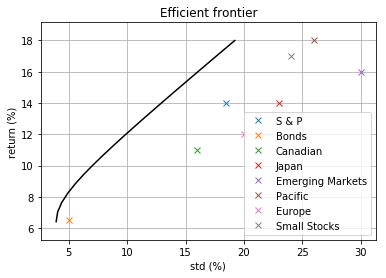The frontier without short sales¶

The Optimize API let's one add any constraint to the calculation of the points in the frontier.

For example:

In :
# vector of expected returns
r = assets['Return (%)'].values

# covariance matrix
sigmas = np.diag(assets['STD (%)'].values)
Q = sigmas @ cov.values @ sigmas

# options
options = {
'number_of_points': 20,
'short': False
}

# prepare data for submitting request to api
data = {
'Q': Q.tolist(),
'r': r.tolist(),
'options': options
}
sol = client.call('frontier', data)

will produce 20 points on the efficient frontier obtained for portfolios for which short sales are not permitted:

In :
frontier = sol['frontier']
m = len(frontier)
mu1 = np.zeros((m,))
var1 = np.zeros((m,))
pos1 = np.zeros((m, n))
for i, e in enumerate(frontier):
point = e['sol']
mu1[i] = e['mu']
var1[i] = point['obj']
pos1[i, :] = point['x']
if point['obj'] is not None:
print('  {}. mu = {:.3f}%, std = {:.3f}% ({})'.format(i+1, mu1[i], math.sqrt(var1[i]), point['status']))
else:
print('  {}. mu = {:.3f}%, std = --- ({})'.format(i+1, mu1[i], point['status']))
1. mu = 6.889%, std = 4.874% (optimal)
2. mu = 7.474%, std = 5.025% (optimal)
3. mu = 8.059%, std = 5.382% (optimal)
4. mu = 8.644%, std = 5.886% (optimal)
5. mu = 9.228%, std = 6.502% (optimal)
6. mu = 9.813%, std = 7.204% (optimal)
7. mu = 10.398%, std = 7.969% (optimal)
8. mu = 10.983%, std = 8.779% (optimal)
9. mu = 11.568%, std = 9.625% (optimal)
10. mu = 12.152%, std = 10.497% (optimal)
11. mu = 12.737%, std = 11.389% (optimal)
12. mu = 13.322%, std = 12.297% (optimal)
13. mu = 13.907%, std = 13.217% (optimal)
14. mu = 14.491%, std = 14.148% (optimal)
15. mu = 15.076%, std = 15.087% (optimal)
16. mu = 15.661%, std = 16.032% (optimal)
17. mu = 16.246%, std = 16.984% (optimal)
18. mu = 16.830%, std = 17.940% (optimal)
19. mu = 17.415%, std = 18.900% (optimal)
20. mu = 18.000%, std = 26.000% (optimal)

Again these points can be combined to produce a plot of the frontier:

In :
rmax = np.max(r)
rmin = np.min((np.min(r), np.min(mu0)))
dr = rmax - rmin
colors = np.array(plt.rcParams['axes.prop_cycle'].by_key()['color'])
In :
# plot frontier
ylim = ((rmin-0.1*dr), (rmax+0.1*dr))

for i, label in enumerate(assets['Label'].values):
plt.plot(np.sqrt(Q[i,i]), r[i], 'x', color=colors[np.mod(i, len(colors))])
plt.plot(np.sqrt(var0), mu0, 'k--')
plt.plot(np.sqrt(var1), mu1, 'k')
plt.xlabel('std (%)')
plt.ylabel('return (%)')
plt.title('Efficient frontier')
plt.ylim(ylim)
plt.legend(assets['Label'].values)
plt.grid()
plt.show()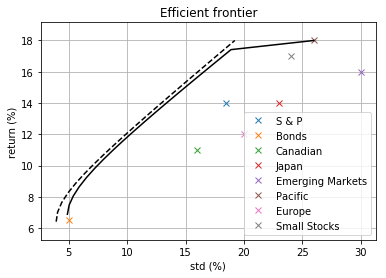We have combined in this plot also the standard frontier with short sales (dashed) for comparison.

The frontier with general constraints¶

All options available for calculation of optimal portfolios also apply to the frontier.

For example, the requirement of no short sales plus at least 50% on S & P and Bonds can be applied to the frontier as follows:

In :
# options
options = {
'number_of_points': 20,
'short': False
}

# prepare data for submitting request to api
data = {
'Q': Q.tolist(),
'r': r.tolist(),
'mu': mu,
'labels': assets['Label'].tolist(),
'constraints': [{'label': 's&p_bonds', 'set': ['S & P', 'Bonds'], 'bounds': [.5, np.inf]}],
'options': options
}
sol = client.call('frontier', data)
In :
frontier = sol['frontier']
m = len(frontier)
mu2 = np.zeros((m,))
var2 = np.zeros((m,))
pos2 = np.zeros((m, n))
for i, e in enumerate(frontier):
point = e['sol']
mu2[i] = e['mu']
var2[i] = point['obj']
pos2[i, :] = point['x']
if point['obj'] is not None:
print('  {}. mu = {:.3f}%, std = {:.3f}% ({})'.format(i+1, mu2[i], math.sqrt(var2[i]), point['status']))
else:
print('  {}. mu = {:.3f}%, std = --- ({})'.format(i+1, mu2[i], point['status']))
1. mu = 6.889%, std = 4.874% (optimal)
2. mu = 7.474%, std = 5.025% (optimal)
3. mu = 8.059%, std = 5.382% (optimal)
4. mu = 8.644%, std = 5.886% (optimal)
5. mu = 9.228%, std = 6.502% (optimal)
6. mu = 9.813%, std = 7.204% (optimal)
7. mu = 10.398%, std = 7.969% (optimal)
8. mu = 10.983%, std = 8.779% (optimal)
9. mu = 11.568%, std = 9.625% (optimal)
10. mu = 12.152%, std = 10.519% (optimal)
11. mu = 12.737%, std = 11.487% (optimal)
12. mu = 13.322%, std = 12.497% (optimal)
13. mu = 13.907%, std = 13.541% (optimal)
14. mu = 14.491%, std = 14.611% (optimal)
15. mu = 15.076%, std = 15.702% (optimal)
16. mu = 15.661%, std = 16.811% (optimal)
17. mu = 16.246%, std = --- (infeasible)

Note how the additional constraints lead to infeasible portfolios for higher levels of return.

This frontier's graphical representation can be calculated as in:

In :
rmax = np.max(r)
rmin = np.min((np.min(r), np.min(mu0)))
dr = rmax - rmin
colors = np.array(plt.rcParams['axes.prop_cycle'].by_key()['color'])
In :
# plot frontier
ylim = ((rmin-0.1*dr), (rmax+0.1*dr))

for i, label in enumerate(assets['Label'].values):
plt.plot(np.sqrt(Q[i,i]), r[i], 'x', color=colors[np.mod(i, len(colors))])
plt.plot(np.sqrt(var0), mu0, 'k--')
plt.plot(np.sqrt(var1), mu1, 'k:')
plt.plot(np.sqrt(var2), mu2, 'k')
plt.xlabel('std (%)')
plt.ylabel('return (%)')
plt.title('Efficient frontier')
plt.ylim(ylim)
plt.legend(assets['Label'].values)
plt.grid()
plt.show()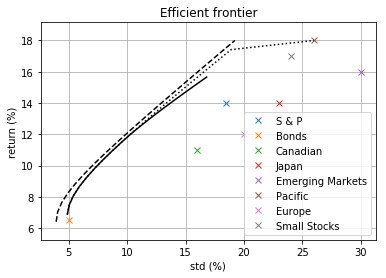A strongly constrained frontier¶

Consider now the cashflow problem we discussed above in which one would like to invest additional funds to improve their returns without selling any assets. The corresponding frontier can be obtained as follows:

In :
# initial portfolio
x0 = assets['Initial (%)'].values/100

# cashflow
cashflow = 20/100

# options
options = {
'number_of_points': 20,
'sell': False
}

# prepare data for submitting request to api
data = {
'Q': Q.tolist(),
'r': r.tolist(),
'x0': x0.tolist(),
'cashflow': cashflow,
'options': options
}
sol = client.call('frontier', data)

Note that in this case many returns lead to infeasible portfolios, and the efficient frontier covers a much smaller range of returns:

In :
frontier = sol['frontier']
m = len(frontier)
mu3 = np.zeros((m,))
var3 = np.zeros((m,))
pos3 = np.zeros((m, n))
for i, e in enumerate(frontier):
point = e['sol']
mu3[i] = e['mu']
var3[i] = point['obj']
pos3[i, :] = point['x']
if point['obj'] is not None:
print('  {}. mu = {:.3f}%, std = {:.3f}% ({})'.format(i+1, mu3[i], math.sqrt(var3[i]), point['status']))
else:
print('  {}. mu = {:.3f}%, std = --- ({})'.format(i+1, mu3[i], point['status']))
1. mu = 11.708%, std = 10.331% (optimal)
2. mu = 12.039%, std = 10.761% (optimal)
3. mu = 12.371%, std = 11.226% (optimal)
4. mu = 12.702%, std = 11.711% (optimal)
5. mu = 13.033%, std = 12.202% (optimal)
6. mu = 13.364%, std = 12.699% (optimal)
7. mu = 13.695%, std = --- (infeasible)

as visualized in the following plot:

In :
rmax = np.max(r)
rmin = np.min((np.min(r), np.min(mu0)))
dr = rmax - rmin
colors = np.array(plt.rcParams['axes.prop_cycle'].by_key()['color'])
In :
# plot frontier
ylim = ((rmin-0.1*dr), (rmax+0.1*dr))

for i, label in enumerate(assets['Label'].values):
plt.plot(np.sqrt(Q[i,i]), r[i], 'x', color=colors[np.mod(i, len(colors))])
plt.plot(np.sqrt(var0), mu0, 'k--')
plt.plot(np.sqrt(var1), mu1, 'k:')
plt.plot(np.sqrt(var2), mu2, 'k-.')
plt.plot(np.sqrt(var3), mu3, 'k')
plt.xlabel('std (%)')
plt.ylabel('return (%)')
plt.title('Efficient frontier')
plt.ylim(ylim)
plt.legend(assets['Label'].values)
plt.grid()
plt.show()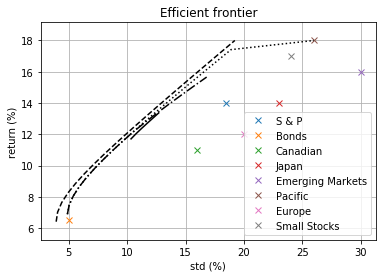Zooming in:

In :
# plot frontier
ylim = (11.5, 13.5)
xlim = (9, 13)

for i, label in enumerate(assets['Label'].values):
plt.plot(np.sqrt(Q[i,i]), r[i], 'x', color=colors[np.mod(i, len(colors))])
plt.plot(np.sqrt(var0), mu0, 'k--')
plt.plot(np.sqrt(var1), mu1, 'k:')
plt.plot(np.sqrt(var2), mu2, 'k-.')
plt.plot(np.sqrt(var3), mu3, 'k')
plt.xlabel('std (%)')
plt.ylabel('return (%)')
plt.title('Efficient frontier')
plt.ylim(ylim)
plt.xlim(xlim)
plt.grid()
plt.show()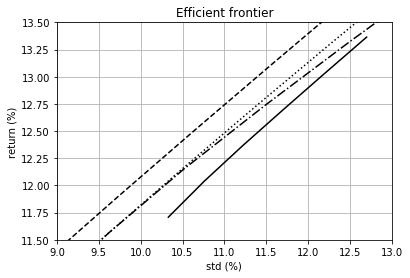helps to visualize the three frontiers.

Another interesting visualizion is that of the resulting trades. The following plot shows the frontier, the corresponding positions as a percentage of the total holdings and the corresponding trades as a percentage of the cashflow.

In :
mu = mu3
var = var3
pos = pos3
trades = (pos - x0) / cashflow

# plot frontier
plt.figure(figsize=(10, 10))

ax = plt.subplot(3, 1, 1)
for i, label in enumerate(assets['Label'].values):
plt.plot(r[i], np.sqrt(Q[i,i]), 'x', color=colors[np.mod(i, len(colors))])
ax.plot(mu0, np.sqrt(var0), 'k--')
ax.plot(mu1, np.sqrt(var1), 'k:')
ax.plot(mu2, np.sqrt(var2), 'k-.')
ax.plot(mu, np.sqrt(var), 'k')
ax.set_ylabel('std (%)')
ax.set_title('Efficient frontier')
ax.set_xlim(ylim)
ax.set_ylim(xlim)
ax.grid()

ax = plt.subplot(3, 1, 2)
ax.plot(mu, 100*pos)
ax.set_ylabel('positions')
ax.set_title('Positions')
ax.set_xlim(ylim)
ax.legend(assets['Label'].values)
ax.grid()

ax = plt.subplot(3, 1, 3)